Unsupervised Cross-domain Image Generation也称为Domain Transfer Network简称为DTN， 作为较早利用GAN思想实现跨模态图像的生成，对于后期的工作有很好的指导和借鉴。文章巧妙地将模态间的的特征做了处理，使得源域提取的特征在模型 作用下趋于目标域的特征，从而实现模态上的转换。

# 论文引入

DTN采用重构源域与目标域的特征空间误差来缩小域之间的特征差异，最后实现较好的统一从而生成上利用特征空间来生成目标域的图像，我们后面会详细说明。 总结一下DTN一文的优势：

• 较早的提出用GAN实现跨模态的生成
• 特征误差的巧妙设计
• 利用目标域的重构加强判别器的能力，从而实现更好的生成
• 实现了无监督下的跨模态生成

# DTN模型

DTN模型的结构框图是很清晰的，我们一起来看一下：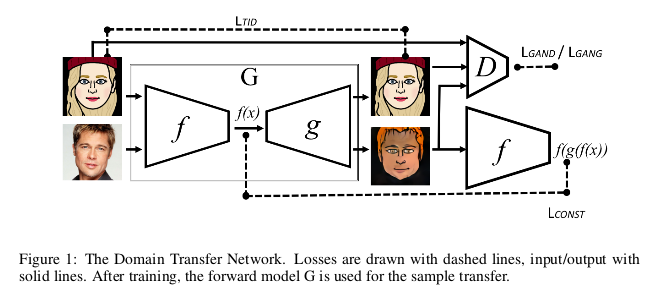$\mathcal L_D = - \mathbb E_{x \in s}[log(1 - D(g(f(x))))]$ $\mathcal L_G = - \mathbb E_{x \in s}[log D(g(f(x)))]$

$\mathcal L_{const} = \sum_{x \in s} d(f(x), f(g(f(x))))$

$\mathcal L_D = - \mathbb E_{x \in t}[log D(x)] - \mathbb E_{x \in t}[log(1 - D(g(f(x))))]$ $\mathcal L_G = - \mathbb E_{x \in t}[log D(g(f(x)))]$

$\mathcal L_{TID} = \sum_{x \in t} d_2(x, G(x))$

$\mathcal L_{TV}(z) = \sum_{i,j}((z_{i,j+1} - z_{ij})^2 + (z_{i+1,j} - z_{ij})^2)^{\frac{B}{2}}$

# DTN实验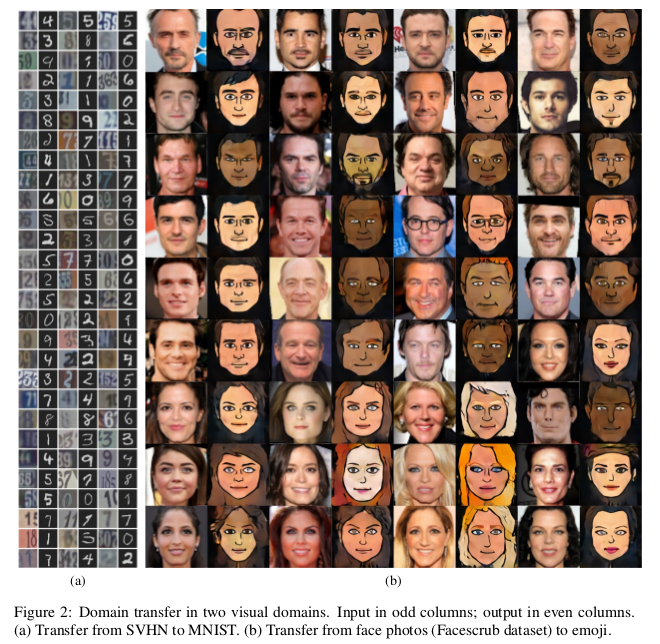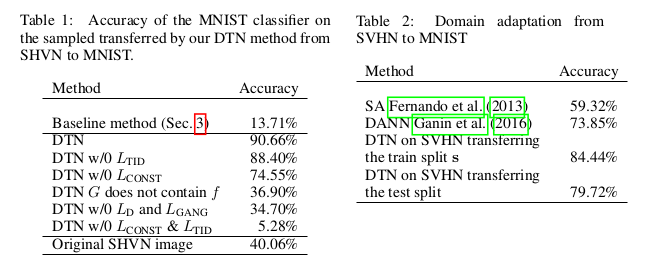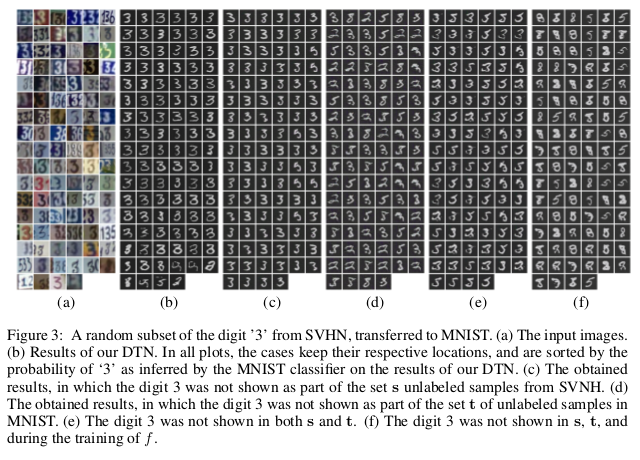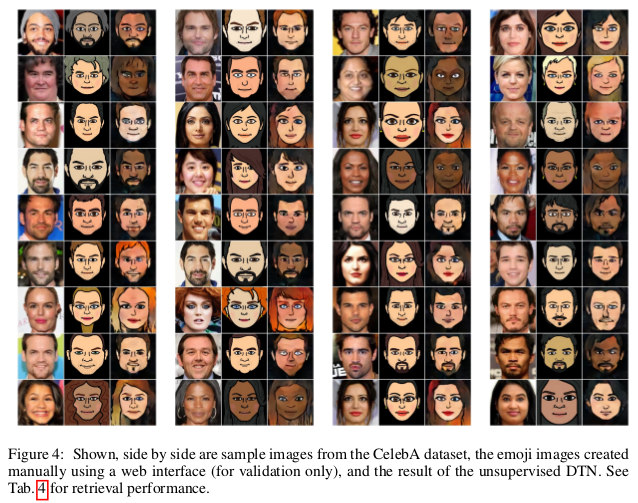DTN存在的一大问题就是生成上的不对称，即有人脸到卡通的域转换可以实现，但是由卡通到人脸则是一个很大的挑战。一个说明是人脸的信息量大，特征空间较为复杂， 仅仅通过特征误差很难使得卡通特征很好的拟合人脸，所以DTN的局限性也正是体现在这里：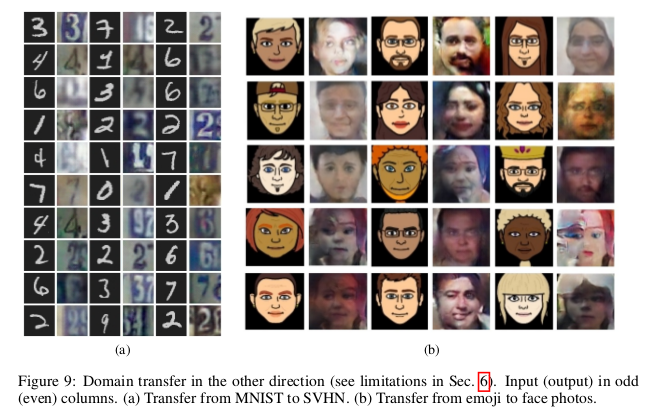# 总结

DTN巧妙设计特征误差实现了模态域间的转换，当目标域的信息量小于源域的时候，通过模型的训练可以很好地实现源域到目标域的生成。但是DTN的一大缺点就是 当目标域的信息量大于源域的时候，此时，模型在实现模态间转换上是不对称的，这也是需要改进的一个地方。